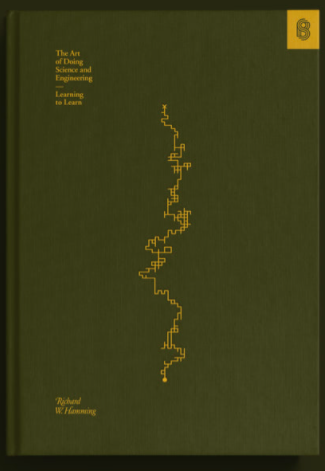# Informed, Random Walk

## Background

Stripe Press published a beautiful copy of “The Art of Doing Science and Engineering” by Richard Hamming:Aside from being a wonderful read, the cover of this book (which is an apt analogy for the learning process) features an intriguing pattern. As I was reading the book while traveling, I was nerd sniped into designing an algorithm which would make such a pattern. This post is an explication of my solution.

## An Informed, Random Walk

Such a pattern could be created from what I am calling an “Informed, Random Walk”. The walk is “informed” because it contains feedback about each step and “random” because each step of the walk is randomly chosen.

### The Context

Imagine we are standing on a point on a 2D plain. We are trying to move from our current location (whose coordinates are unknown to us) to a specific, target location (whose coordinates are also unknown to us). Imagine that we are only allowed to take steps in cardinal directions and, after taking a step, we will be told whether we are closer to the target location.

### The Algorithm

What algorithm would help us get to the target location as directly as possible? A basic algorithm is:

1. Randomly choose a direction and take a step in that direction
2. Determine whether the step brings us closer to the target
3. If the step took us closer to the target, make it more likely to choose that direction in the future, otherwise make it less likely

### The Data Structure

Perhaps equally as important as the algorithm is the data structure used to track the steps we’ve taken and their results. For this post, I’ve written the algorithm using ClojureScript so I’m using a map which looks like:

``````{:North 1 :East 1 :South 2 :West 3}
``````

The keys of the map are the possible directions and the values are the weights for that direction.

At step 1 in the algorithm (when we randomly choose a direction), we use the weights to determine the likelihood of a direction being chosen. For example, given the map above, we would randomly choose a direction where `:South` is two times are likely to be chosen as `:North` and `:East` and where `:West` is three times more likely than `:North` and `:East`. The way this is actually implemented, we take the map of directions and their weights and generate an array where each direction is repeated as many times as the weight. So, using the map above as an example, we would have:

``````[:North :East :South :South :West :West :West]
``````

We then randomly choose one of these to determine in which direction we will take a step.

At step 3 of the algorithm (where we record feedback about the step), we update the map with the directions in it according to whether or not the step brought us closer to the target. So say our direction map is:

``````{:North 1 :East 1 :South 2 :West 3}
``````

We take a step `:North` and this takes us closer to the target. So, we now want to make it more likely to take another step `:North`, so we increment the value for `:North`:

``````{:North 2 :East 1 :South 2 :West 3}
``````

This is the interesting part of the whole algorithm! Given a direction map, the direction of the most recent step, and whether or not that step brought you closer to the target: How do you update the direction map to best approach the destination?

### The Code

Below is interactive code that sets the context for this problem. Some of the less important functionality is hidden, but feel free to edit what is visible. If you scroll below the code blocks, you will see an informed, random walk based on the code. Enjoy exploring!

Here are some of the underlying functions used to walk around the canvas. For starters, I recommend you skip this code block to the next, more interesting one below.
``````
(def step-length 10)
(def max-step-count 1000)
(def dirs {:North 2 :East 2 :South 2 :West 2})

(defn make-weighted-list [dirs]
(flatten (map #(repeat (max (second %) 0) (first %)) dirs)))

(defn get-dir [dirs]
(rand-nth (make-weighted-list dirs)))

(defn get-next-loc [current dir]
(case dir
:North [(current 0) (- (current 1) step-length)]
:East [(+ (current 0) step-length) (current 1)]
:South [(current 0) (+ (current 1) step-length)]
:West [(- (current 0) step-length) (current 1)]))

(defn opposite-dir [dir]
(case dir
:North :South
:East :West
:South :North
:West :East))

(defn walk [start dirs]
(loop [current start
dist (get-dist start)
dirs dirs
steps [start]]
(if (or (= current target) (> (count steps) max-step-count))
steps
(let [dir (get-dir dirs)
next-loc (get-next-loc current dir)
new-dist (get-dist next-loc)
closer? (neg? (- new-dist dist))
new-dirs (update-dirs dirs dir closer?)]
(recur next-loc new-dist new-dirs (conj steps next-loc))))))

(defn draw []
(setup start)
(let [steps (walk start dirs)
success? (= (last steps) target)]
(print-output (count (draw-steps steps)) success?)))
``````
This is the interesting stuff! The `update-dirs` function is the one we use to update the weights given to each of the directions (thus, making it more or less likely for the walker to walk in that direction). If the walker doesn't find the target in 1,000 steps, it will stop (but you can change this by changing the `max-step-count` variable in the code block above). Feel free to edit the code below to see how this changes the walker's behaviour:
``````
(def start [50 50])
(def target [300 300])
(def dir-increment 1)

(defn update-dirs [dirs dir closer?]
(if (true? closer?)
(update-in dirs [dir] #(+ % dir-increment))
(update-in dirs [dir] #(max 1 (- % dir-increment)))))
```
```

The default `update-dirs` function is pretty bad (it rarely finds the target).

What if you update it to:

``````(defn update-dirs [dirs dir closer?]
(if (true? closer?)
(update-in dirs [dir] #(+ % dir-increment))
(update-in (update-in dirs [dir] #(- % dir-increment)) [(opposite-dir dir)] #(+ % dir-increment))))
``````